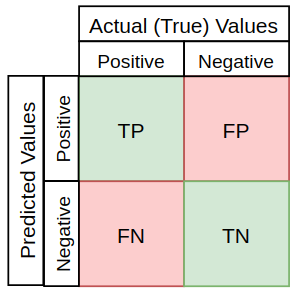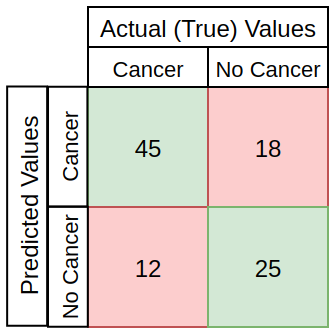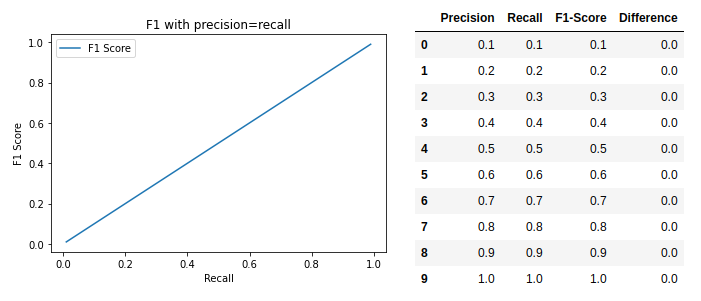Precision, recall and F1-scores are used to evaluate classification models in machine learning.

The basis of the evaluation is usually the overall model accuracy which is the number of correct predictions divided by the size of the dataset. Accuracy only considers true positives and negatives.

## Precision and recall §

Precision measures how many positive predictions were actually correct (true positives) and is calculated by dividing the number of true positives by the number of all positive predictions (true and false).

→ What fraction of positive predictions where actually correct?

Recall (also called Sensitivity in Statistics) is used to evaluate how many positive cases were predicted correctly. It is calculated by dividing true positives by the sum of all true positives and false negatives.

→ What fraction of all positive instances in the data have I predicted to be positive?

Specificity measures true negatives.

→ What fraction of correctly predicted negatives are actual negatives?

Example: Cancer PatientsPrecision is the number of correctly predicted people with cancer over the number of all people the model predicted to have cancer. → I predicted that a total of 45 + 18 people have cancer but only 45 of those actually have cancer.

Recall is the number of all true positive cases in data over the number of correctly predicted cases. → There is a total of 45 + 12 people who really have cancer but I only accounted for 45 of them.

## F1 Score §

The F1-score combines precision and recall. It’s represented by the harmonic mean of the two measurements.

It’s supposed to provide a balanced overview.

If recall = precision, then